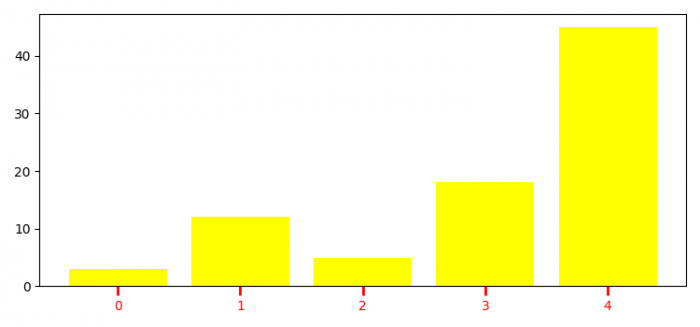# How to customize X-axis ticks in Matplotlib?

To customize X-axis ticks in Matplotlib, we can change the ticks length and width.

## Steps

• Set the figure size and adjust the padding between and around the subplots.
• Create lists for height, bars and y_pos data points.
• Make a bar plot using bar() method.
• To customize X-axis ticks, we can use tick_params() method, with color=red, direction=outward, length=7, and width=2.
• To display the figure, use show() method.

## Example

import numpy as np
import matplotlib.pyplot as plt

plt.rcParams["figure.figsize"] = [7.50, 3.50]
plt.rcParams["figure.autolayout"] = True

height = [3, 12, 5, 18, 45]
bars = ('A', 'B', 'C', 'D', 'E')
y_pos = np.arange(len(bars))

plt.bar(y_pos, height, color='yellow')
plt.tick_params(axis='x', colors='red', direction='out', length=7, width=2)

plt.show()

## Output